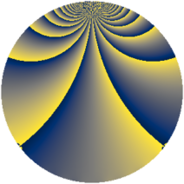# Properties

 Label 1210.2.sLevel $1210$ Weight $2$ Character orbit 1210.s Rep. character $\chi_{1210}(31,\cdot)$ Character field $\Q(\zeta_{55})$ Dimension $1760$ Sturm bound $396$

# Related objects

## Defining parameters

 Level: $$N$$ $$=$$ $$1210 = 2 \cdot 5 \cdot 11^{2}$$ Weight: $$k$$ $$=$$ $$2$$ Character orbit: $$[\chi]$$ $$=$$ 1210.s (of order $$55$$ and degree $$40$$) Character conductor: $$\operatorname{cond}(\chi)$$ $$=$$ $$121$$ Character field: $$\Q(\zeta_{55})$$ Sturm bound: $$396$$

## Dimensions

The following table gives the dimensions of various subspaces of $$M_{2}(1210, [\chi])$$.

Total New Old
Modular forms 8080 1760 6320
Cusp forms 7760 1760 6000
Eisenstein series 320 0 320

## Trace form

 $$1760q - 2q^{2} - 4q^{3} + 44q^{4} + 6q^{6} - 2q^{8} - 432q^{9} + O(q^{10})$$ $$1760q - 2q^{2} - 4q^{3} + 44q^{4} + 6q^{6} - 2q^{8} - 432q^{9} + 8q^{10} + 8q^{11} + 18q^{12} + 52q^{13} + 42q^{14} + 56q^{15} + 44q^{16} - 8q^{17} - 16q^{18} + 14q^{19} - 48q^{21} - 10q^{22} - 32q^{23} + 6q^{24} + 44q^{25} + 20q^{26} + 26q^{27} + 40q^{29} - 4q^{30} + 96q^{31} + 8q^{32} - 34q^{33} - 20q^{34} - 8q^{35} + 42q^{36} + 24q^{37} - 12q^{38} + 32q^{39} + 20q^{40} + 30q^{41} + 24q^{42} - 12q^{43} - 2q^{44} + 8q^{46} - 12q^{47} - 4q^{48} + 114q^{49} - 2q^{50} + 56q^{51} - 12q^{52} + 140q^{53} - 16q^{54} + 8q^{55} - 12q^{56} - 48q^{57} + 72q^{58} - 46q^{59} - 8q^{60} + 24q^{61} - 4q^{62} + 44q^{63} + 44q^{64} + 20q^{65} - 6q^{66} + 24q^{67} - 8q^{68} + 28q^{69} + 16q^{71} + 14q^{72} + 112q^{73} + 4q^{74} + 6q^{75} + 72q^{76} + 88q^{77} - 32q^{78} + 132q^{79} - 430q^{81} + 114q^{82} + 34q^{83} + 12q^{84} + 104q^{85} - 2q^{86} + 48q^{87} + 76q^{88} - 8q^{89} + 62q^{90} + 246q^{91} + 160q^{92} + 196q^{93} + 114q^{94} - 4q^{96} + 186q^{97} + 184q^{98} - 76q^{99} + O(q^{100})$$

## Decomposition of $$S_{2}^{\mathrm{new}}(1210, [\chi])$$ into newform subspaces

The newforms in this space have not yet been added to the LMFDB.

## Decomposition of $$S_{2}^{\mathrm{old}}(1210, [\chi])$$ into lower level spaces

$$S_{2}^{\mathrm{old}}(1210, [\chi]) \cong$$ $$S_{2}^{\mathrm{new}}(121, [\chi])$$$$^{\oplus 4}$$$$\oplus$$$$S_{2}^{\mathrm{new}}(242, [\chi])$$$$^{\oplus 2}$$$$\oplus$$$$S_{2}^{\mathrm{new}}(605, [\chi])$$$$^{\oplus 2}$$